## Ordinary Differential Equation--First-Order

Given a first-order Ordinary Differential Equation(1)

if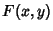can be expressed using Separation of Variables as(2)

then the equation can be expressed as(3)

and the equation can be solved by integrating both sides to obtain(4)

Any first-order ODE of the form(5)

can be solved by finding an Integrating Factor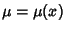such that(6)

Dividing through by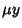yields(7)

However, this condition enables us to explicitly determine the appropriatefor arbitrary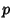and. To accomplish this, take(8)

in the above equation, from which we recover the original equation (5), as required, in the form(9)

But we can integrate both sides of (8) to obtain(10)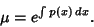(11)

Now integrating both sides of (6) gives(12)

(withnow a known function), which can be solved forto obtain(13)

whereis an arbitrary constant of integration.

Given anth-order linear ODE with constant Coefficients(14)

first solve the characteristic equation obtained by writing(15)

and setting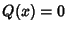to obtain theComplex Roots.(16)(17)

Factoring gives the Roots,(18)

For a nonrepeated Real Root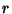, the corresponding solution is(19)

If a Real Rootis repeatedtimes, the solutions are degenerate and the linearly independent solutions are(20)

Complex Roots always come in Complex Conjugate pairs,. For nonrepeated Complex Roots, the solutions are(21)

If the Complex Roots are repeatedtimes, the linearly independent solutions are(22)

Linearly combining solutions of the appropriate types with arbitrary multiplicative constants then gives the complete solution. If initial conditions are specified, the constants can be explicitly determined. For example, consider the sixth-order linear ODE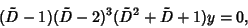(23)

which has the characteristic equation(24)

The roots are 1, 2 (three times), and, so the solution is(25)

If the original equation is nonhomogeneous (), now find the particular solutionby the method of Variation of Parameters. The general solution is then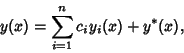(26)

where the solutions to the linear equations are,, ...,, andis the particular solution.

See also Integrating Factor, Ordinary Differential Equation--First-Order Exact, Separation of Variables, Variation of Parameters

References

Arfken, G. Mathematical Methods for Physicists, 3rd ed. Orlando, FL: Academic Press, pp. 440-445, 1985.